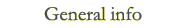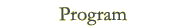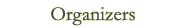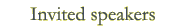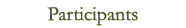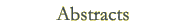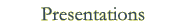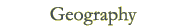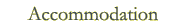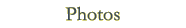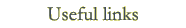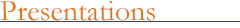Hans Schneider ILAS Lecture Vladimir Müller: Circles in the spectrum and numerical ranges Invited talks Alexander Guterman: Krauter conjecture on permanents is true Dijana Ilišević: On projections arising from isometries with finite spectrum on Banach spaces Thomas Laffey: On the lengths of some generating sets of matrix algebras Chi-Kwong Li: Matrix problems in quantum information science Helena Šmigoc: An equivalence result in the symmetric nonnegative inverse eigenvalue problem Contributed talks Anita Buckley: Indecomposable matrices defining plane cubics Mikhail Budrevich: Extremal non-convertible fully indecomposable (0,1)-matrices Yorick Hardy: Some open questions about Kronecker quotients Damjana Kokol Bukovšek: Linear spaces of symmetric nilpotent matrices Zhening Li: Bounds on tensor norms via tensor partitions Olga Markova: Length realizability problem for pairs of quasi-commuting matrices Blaž Mojškerc: Jordan triple product homomorphisms on triangular matrices to and from dimension one Marko Orel: On matrix theory, graph theory, and finite geometry Aljoša Peperko: Inequalities on the spectral radius, operator norm and numerical radius of Hadamard weighted geometric mean of positive kernel operators Bor Plestenjak: Minimal determinantal representations of bivariate polynomials Rajna Rajić: The Birkhoff--James and Roberts orthogonality in C*-algebras Stephan Weis: A variation principle for ground spaces Aljaž Zalar: There are many more positive maps than completely positive maps